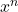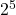A. Express log 96 in terms of log 2 and log 3.

Question

A. Express log 96 in terms of log 2 and log 3.

in progress 0
6 months 2021-07-22T00:13:30+00:00 2 Answers 4 views 0

linear equations

Step-by-step explanation:

5log2 + log3

Step-by-step explanation:

Using the rules of logarithms

logx + logy = log(xy)

log⇔ nlogx

Given

log96

Express 96 as a product of its prime factors

96 =× 3 , then

log96

= log(× 3 )

= log+ log3

= 5log2 + log3